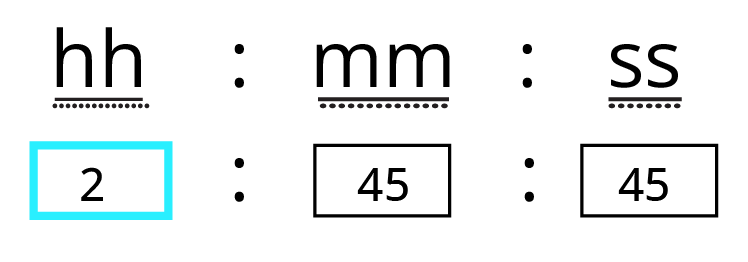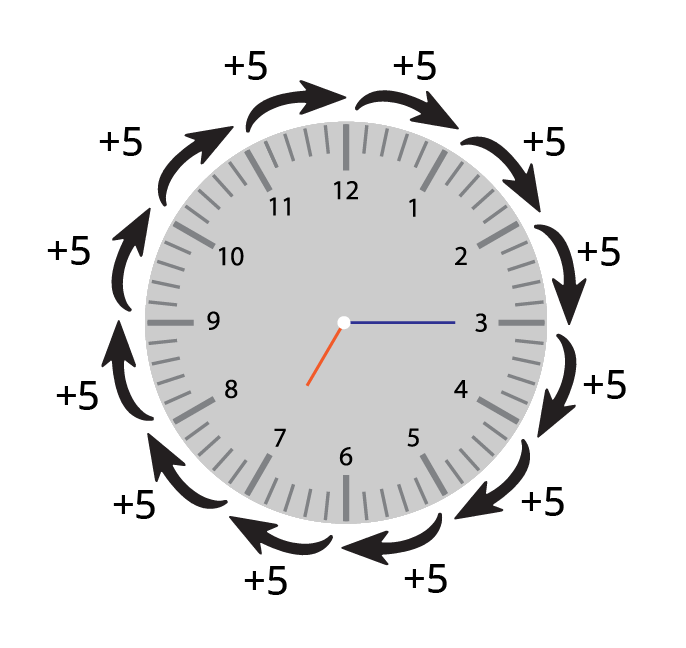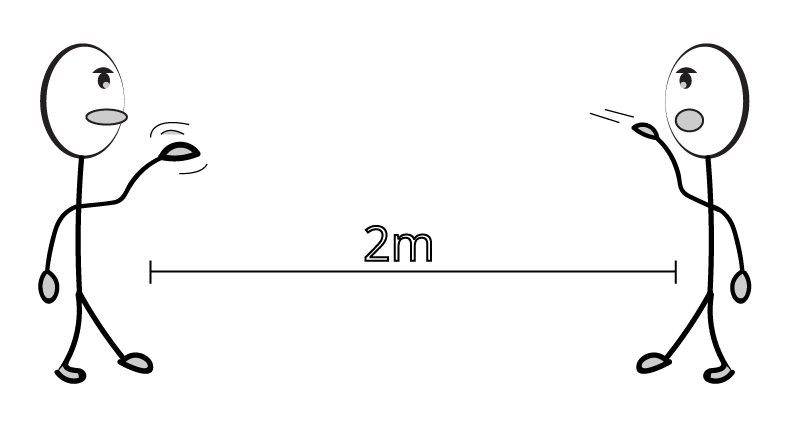Courses
Courses for Kids
Free study material
Free LIVE classes
More

# A Trip to Bhopal Class 4 Notes CBSE Maths Chapter 3 (Free PDF Download)LIVE
Join Vedantu’s FREE Mastercalss

## Free CBSE Class 4 Maths Chapter 3 Revision Notes and Worksheets for Practice

Class 4 is a crucial stage for learning advanced mathematical principles, rules, and formulae to use for solving problems. In this syllabus, Chapter 3 A Trip to Bhopal focuses on teaching students how to use multiplication, addition, and division to find the answers to exercise problems.

The main objective of this chapter is to teach students how to use multiple mathematical operations to solve arithmetic problems. Comprehending the concepts becomes a lot easier when you download and use A Trip to Bhopal revision notes and worksheets.

Last updated date: 27th Sep 2023
Total views: 144k
Views today: 2.44k

## Revision Notes Class 4 Mathematics Chapter 3 - Trip to Bhopal Notes

### Time

• The period in which something happens is called time.

• Time is measured in hours, minutes, or seconds.

• We have seen a clock in our homes, it has 2 hands hour hand and a minute hand.

• Time can be represented as 1:00:00 am or 1:00:00 pm.Time

• Here, hh represents the hour, mm represents minutes, and ss represents seconds.

• Example: Tina wakes up at 7:00:00 am. Get ready and finish her breakfast by 7:45:04 am. And goes to school at 8:00:15 am. In the example, everything that is bold tells about time.

### Sequence of Events

• If the things or events happen continuously like in the above example then it is considered to be a sequence of events.

• We have 24 hours in a day, 60 minutes in an hour and 60 seconds in a minute.

• The clock includes numbers from 1 to 12. And when the minute hand that is the bigger hand is moved from ‘1’ to ‘2’ we say that 5 minutes have been completed.

• This means that when the minute clock moves to the next number we can add “5” to find the time.Sequence of time

Solved Example 1: The students are going on a trip to Bhopal. They left at place ‘A’ at 8:15 am. If it takes 2 hours to reach Bhopal, then at what time do they reach Bhopal?

Ans: Given, Students left at 8:15 am. And it will take 2 hours to reach Bhopal.

So, if we add 2 hours to 8:15, we will get the final time.

We can represent one hour as 1:00:00 and 8:15 am as 8:15:00 am

1:00:00 + 8:15:00 am = 9:15:00 am

If we add one more hour, we get the final time as

9:15:00 am + 1:00:00

= 10:15:00 am

### Distance

• The length between two points is called distance.

• The distance can be measured in terms of meters, centimetres or kilometres.Distance between two people is 2m

### Units of Distance:

 Units Uses Centimetre - cm Used to measure shorter distances by using scales, such as in your Maths class. Metre - m (1 m = 100 cm) Used to measure cloth, used in shops. Kilometre - km (1 km = 1000 m) Used to measure long-distance, roads, bridges,

Solved example 1: Convert the following

a. 25 m = ? cm

Ans: From the above table we know that 1 m = 100 cm. Hence 25 m will be 25 multiplied by 100 cm,

25 × 100 = 2500 cm

b. 2 km = ? m

Ans: From the above table we know that 1 km = 1000 m. Hence 2 km will be 2 multiplied by 1000 m,

2 ×1000 = 2000 m

Solved example 2: On the way to the trip, the students found the flowing Narmada river under the bridge. If the river is 800 m wide, then the bridge will be 500 metres. Convert 500 metres into kilometres.

Ans: We know that 1 km = 1000 m if we divide 1000 by 2, then the result will be 500. This means that

1km=100m/2=500m

We can rewrite it as,

1km=1/2× 1000m =500m

This means that half of a kilometre is 500 metres.

Hence we can write 500 m as half a kilometre.

Solved example 3: Suppose you are going for a trip, there you found a bridge. If we can keep 25 buses in a line on the bridge, then the length of the bridge will be? (Hint: length of 1 bus = 15 metres)

Ans: As per the question we can align 25 buses in a line, and 1 bus = 15 m.

Hence 25 multiplied by 15 gives us the length of the bridge.

25×15 = 375 metres.

### Price

• The value that is given to a thing is called price.

• We can pay price in terms of rupees, dollars, cents, etc based on the country we are living in.

### Solved examples: Use the table below to answer the questions:

 Boat Name Price for the Ticket Trip time Double-decker 30 Rupees 45 minutes Paddleboat 15 Rupees 30 minutes Motorboat 25 Rupees 20 minutes Boat with oars 15 Rupees 45 minutes

Solved example 1: If 2 students choose Paddle-boat, then what is the total price paid by students?

Ans: From the given data, 2 students choose a Paddle-boat. And the price for boating is 15 rupees. Hence the total price will be the number of students multiplied by 15.

2×15 = 30 rupees

Solved example 2: Five of the students choose a Double-decker boat, what is the total trip time spent by them?

Ans: From the table of Price-list it is mentioned that the students who choose a Double-decker boat can spend 45 minutes. Hence from the above-given data, 5 students chose a Double-decker boat and spent 45 minutes enjoying the boating.

### Practise Questions

1. Convert the following

a) 60 m = ? cm

b) 5 km = ? m

2. Tina went shopping and bought 2 shirts, a pair of pants, and 1 pair of earrings. She paid 450 rupees for 2 shirts, 600 for a pant, and 150 for a pair of earrings. What is the total price paid by Tina?

Ans 1: a. 6000 cm

b. 5000 m

Ans 2: 1,200 rupees

## Importance of CBSE Class 4 Maths Chapter 3 A Trip to Bhopal

The Class 4 Maths syllabus is important for the students as the chapters introduce complex mathematical principles derived from the simpler ones studied in previous years. Here is a list of points that define its importance.

• Chapter 3 focuses on teaching how to use the mathematical operations of addition, multiplication, subtraction and division.

• The concepts are taught in a story mode where students will learn and remember them easily.

• The situations are described using simple information related to a bus journey. Students will use their skills in mathematical operations to find the answers.

• The use of different physical quantities and their units also make Class 4 maths Chapter 3 quite crucial. Students will recall using the units and converting them to find the right answers.

• This chapter provokes the logical reasoning and problem-solving skills of students based on real-life events. The incidents of a trip enable are designed in the form of exercise problems.

## Benefits of Vedantu’s A Trip to Bhopal Class 4 Revision Notes and Worksheets

• The revision notes comprise the A Trip to Bhopal summary of all the sections. These summaries are explained by using simple language so that students can understand them easily.

• The use of different physical quantities and their various units will help students to recall what they have studied in the previous years. They can use the revision notes to use mathematical operations to solve the problems by converting units too.

• You will also be able to compare and find out the smaller and bigger units in this chapter. Use the revision notes to find out how many buses can fit on a bridge or how old are the caves.

• Solving A Trip to Bhopal Class 4 worksheets will also help you to answer questions related to making numbers with given digits. You will also learn how to add, multiply, subtract and divide in a single exercise problem to find the right answer.

## Conclusion

Download the free PDF revision notes and worksheets from Vedantu and have a stress-free studying session to finish this chapter. Learn the ideas and review them more quickly before the exam. To assess your problem-solving abilities, use the Class 4 Mathematics Chapter 3 answer for worksheets. The trip may have been an opportunity for the Class 4 students to witness the rich culture and heritage of Bhopal, visit historical landmarks, and interact with the locals to understand their way of life. Exploring the city's historical sites, trying local cuisine, and experiencing its unique traditions could have left a lasting impact on the young travelers.

## FAQs on A Trip to Bhopal Class 4 Notes CBSE Maths Chapter 3 (Free PDF Download)

1. How studying A Trip to Bhopal will help me understand units?

This story-based Maths chapter will help you understand various physical quantities and measuring units. You will also learn how to apply addition, subtraction, multiplication, and division methods to answer questions in exams.

2. How can I understand the units and solve problems?

Check the units and find out what the question is asking. Focus on how to change the units and use your mathematical skills to answer.

3. How can I solve the exercise questions for Chapter 3 easily?

Download NCERT solutions from Vedantu to check how problems are solved by the experts. Adopt those proven approaches and perform better.

4. How can teachers and parents prepare students for a trip like "A Trip to Bhopal"?

Teachers and parents can prepare students for a trip by providing information about the destination, discussing its historical and cultural significance, and setting learning objectives for the journey.

5. What kind of activities might students engage in during "A Trip to Bhopal"?

During "A Trip to Bhopal," students might visit historical monuments, museums, local markets, and other tourist attractions. They could participate in interactive sessions, cultural programs, or workshops relevant to the destination.